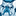# App Development

Announcements
Action-Packed Learning Awaits! QlikWorld 2023. April 17 - 20 in Las Vegas: REGISTER NOW
cancel
Showing results for
Did you mean:Creator III

## Creating an expression with Multiple Variables

I have a dataset as follows:

Type    Decider        Value90       Value180     Value365

C           100             50000          35000           42000

N           450             12000          33000           45000

C           500             21000          24000           41000

C           300             32000          30000           40000

N           200             40000          39000           43000

C           156             37000          46000           39500

Now I also have three Variables, vDecider, vDays and vType. vDays can take values of 90,180 and 365. vType can take values of General,L1 and L2. vInp is a dynamic variable that can take any numeric value.

Let's say vType=General,vDays=90 and vDecider=300, then I'd like to sum the field Value90 where Decider field<=300 and the output should be 159000.(1st+4th+5th+6th fields). Similarly, if vDays=180 then sum Value180 field where Decider<=300 and so on.

Now if vType='L1', vDays=90 and vDecider=300, then I'd like to sum the field Value90 where Type is only C and Decider Field<=300 and the output should be 119000(1st+5th+6th field). Similarly, if vDays=180 then sum Value180 field where Decider<=300 and so on.

If vType='L2', vDays=90 and vDecider=300 then sum the field Value90 where Type is only N and Decider field<=300 and the output should be 40000(5th field only should be selected).Similarly, if vDays=180 then sum Value180 field where Decider<=300 and so on.

I'd like to create an expression for getting a measure as mentioned above.I have tried nested IF loops but they are not working. Any help is much appreciated. TIA!

0 RepliesTags
Community Browser